Impact Factor 3.693 | CiteScore 2.5
More on impact ›

# Frontiers in Chemistry## Original Research ARTICLE

Front. Chem., 02 August 2019 | https://doi.org/10.3389/fchem.2019.00537

# State-Selected Reactivity of Carbon Dioxide Cations (${\text{CO}}_{2}^{+}$) With Methane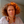Daniela Ascenzi1*,Claire Romanzin2,3,Allan Lopes2,Paolo Tosi1,Jan Žabka4,Miroslav Polášek4,Christopher J. Shaffer5 andChristian Alcaraz2,3
• 1Department of Physics, University of Trento, Trento, Italy
• 2Laboratoire de Chimie Physique, Bât. 350, UMR 8000, CNRS-Univ. Paris-Sud and Paris Saclay, Centre Universitaire Paris-Sud, Orsay, France
• 3Synchrotron SOLEIL, L'Orme des Merisiers, Saint-Aubin—BP 48, Gif-sur-Yvette, France
• 4J. Heyrovský Institute of Physical Chemistry of the Czech Academy of Sciences, Prague, Czechia
• 5Institute of Organic Chemistry and Biochemistry of the Czech Academy of Sciences, Prague, Czechia

The reactivity of ${\text{CO}}_{2}^{+}$ with CD4 has been experimentally investigated for its relevance in the chemistry of plasmas used for the conversion of CO2 in carbon-neutral fuels. Non-equilibrium plasmas are currently explored for their capability to activate very stable molecules (such as methane and carbon dioxide) and initiate a series of reactions involving highly reactive species (e.g., radicals and ions) eventually leading to the desired products. Energy, in the form of kinetic or internal excitation of reagents, influences chemical reactions. However, putting the same amount of energy in a different form may affect the reactivity differently. In this paper, we investigate the reaction of ${\text{CO}}_{2}^{+}$ with methane by changing either the kinetic energy of ${\text{CO}}_{2}^{+}$ or its vibrational excitation. The experiments were performed by a guided ion beam apparatus coupled to synchrotron radiation in the VUV energy range to produce vibrationally excited ions. We find that the reactivity depends on the reagent collision energy, but not so much on the vibrational excitation of ${\text{CO}}_{2}^{+}$. Concerning the product branching ratios (${\text{CD}}_{4}^{+}$/${\text{CD}}_{3}^{+}$/DOCO+) there is substantial disagreement among the values reported in the literature. We find that the dominant channel is the production of ${\text{CD}}_{4}^{+}$, followed by DOCO+ and ${\text{CD}}_{3}^{+}$, as a minor endothermic channel.

## Introduction

The chemistry of the ${\text{CO}}_{2}^{+}$ cation attracts much attention because of the presence of this ion in planetary atmospheres (with particular reference to the Earth and Mars Matta et al., 2013; Tenewitz et al., 2018 as well as in laboratory plasmas for energetic and environmental applications Snoeckx and Bogaerts, 2017). In the latter field, the efficient conversion of greenhouse gases into value-added chemicals is a central topic in current research on renewable and sustainable energies (Wang et al., 2017). In particular, the hydrogenation of CO2 by technologies based on green electricity allows both the storage of renewable energy in value-added compounds and recycling CO2, thus paving the way to decarbonise the energy system. Non-thermal plasmas have been explored for their capability to activate very stable molecules with the potential of achieving a higher energy efficiency compared to purely thermal processes (Scapinello et al., 2016; Martini et al., 2018). To improve the performances and to control the outcome of plasma-based processes, insight into the physical and chemical mechanisms at play is desired. According to a chemical kinetic model of the plasma-based dry reforming (Snoeckx et al., 2013), a key role is played by the reaction of ${\text{CO}}_{2}^{+}$ with CH4. However, as described below, there is considerable uncertainty on the branching ratio, so that a reinvestigation of the reaction is desirable. Also, because vibrationally excited levels of ${\text{CO}}_{2}^{+}$ can be populated in plasmas, this study aims at investigating the effect of the vibrational excitation of the ${\text{CO}}_{2}^{+}$ cation on the reaction with CH4.

Energy, in the form of kinetic or internal motion of the reagents, is the driving force of chemical reactions. However, putting the same amount of energy in a different form (i.e., translational, vibrational, rotational or electronic energy) may affect the reactivity differently. For ion-molecule reactions, some state-selected experiments have shown that for endothermic charge-transfer (CT) processes, vibrational excitation is more effective than translational energy in driving the reactions (Viggiano and Morris, 1996). However, in other cases, the effect of vibrational excitation is more varied (see for example Candori et al., 2003; Boyle et al., 2011; Chang et al., 2012; Bell and Anderson, 2013a,b; Bell et al., 2014 and reference therein).

The effect of the internal excitation of ${\text{CO}}_{2}^{+}$ in reactions with small molecules has been addressed in previous studies. It was found that the vibrational excitation of ${\text{CO}}_{2}^{+}$ increases the reactivity with O2 and NO (Alge et al., 1981; Durup-Ferguson et al., 1983; Derai et al., 1985; Ferguson et al., 1992; Nicolas et al., 2002), while it decreases the rate coefficient for the reaction with H2 (Albritton, 1979; Borodi et al., 2009). However, no previous studies exist in which the ${\text{CO}}_{2}^{+}$ cation is generated with a precise amount of internal energy (i.e., state-selection of a specific vibrational state) and reacted with CH4.

## Previous Studies of the ${\text{CO}}_{2}^{+}$ + CH4 Reaction

The reaction of ${\text{CO}}_{2}^{+}$ with methane in the gas phase has been studied by several groups, with the earliest experimental results dating back to the late 60s (Anicich, 2003). Rate constant and product branching ratio measurements were made using drift techniques, either flow drift tubes (FDT) (Rakshit and Warneck, 1980; Durup-Ferguson et al., 1983) or selected ion flow tube (SIFT) (Smith et al., 1978; Copp et al., 1982), ion cyclotron resonance (ICR) techniques (Huntress et al., 1980) and ion beam methods (Tsuji et al., 1994). Earlier determinations where done using high-pressure mass spectrometry (HPMS) (Harrison and Myher, 1967; Chong and Franklin, 1971; Kasper and Franklin, 1972) and electron space charge traps (SCT) (Ryan and Harland, 1974). The most relevant results are summarized in Table 1.

TABLE 1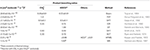Table 1. Summary of existing experimental determinations of rate constants and branching ratios for the reaction of ${\text{CO}}_{2}^{+}$ with CH4.

It has been shown that the reaction proceeds at thermal energy with a rate constant close to the Langevin collision rate constant kL = 1.1 × 10−9 cm3·molecule−1·s−1 (Durup-Ferguson et al., 1983). There is fair agreement (within the experimental errors) among the total rate constants measured at thermal energies (in the range 280–340 K for the data reported in Table 1), with the exception of the HPMS study by Kasper and Franklin (1972), that gives a rate constant value more than a factor two higher than the others. The values for the branching ratios are quite scattered, with HOCO+ being dominant in all studies except (Durup-Ferguson et al., 1983), where the CT is the only observed channel.

## Materials and Methods

The experiments have been performed using the CERISES apparatus, an associated experiment to the SOLEIL synchrotron radiation facility. Since the set-up was described in details previously (see Alcaraz et al., 2004; Cunha de Miranda et al., 2015), only the most relevant details will be given here. CERISES is a guided ion beam tandem mass spectrometer composed of two octopoles located between two quadrupole mass spectrometers in a Q1-O1-O2-Q2 configuration that permits investigation of bi-molecular reactions of mass-selected ions. By measuring the yields of parent- and product-ions, absolute reaction cross sections, branching ratios and product velocity distributions as a function of the collision energy are derived.

Vibrational state selection of ${\text{CO}}_{2}^{+}$ is performed via the Threshold Photoelectron Photoion Coincidence (TPEPICO) method (Baer and Guyon, 1986) using the ion source of CERISES and the DESIRS beamline. The undulator based DESIRS beamline (Nahon et al., 2012) provides tunable radiation in the vacuum ultraviolet (VUV) range from about 5 eV to 40 eV. Photons at the desired wavelength are selected and scanned simultaneously with the undulator peak energy by a normal incidence monochromator equipped with a low dispersion uncoated SiC grating (200 grooves/mm) optimized to provide photon flux in the 1012 photon/s to 1013 photon/s range with an energy resolution down to 1 meV in the 5 eV to 20 eV range. In the present experiments, the photon energies (Ephot) required to produce the parent ion by photoionisation are in the range 13.7–18.3 eV. Depending on the operation mode, the monochromator slits were set in the range 25 to 400 μm, corresponding, at these photon energies, to a resolution of 3 to 44 meV.

In the TPEPICO mode, the ${\text{CO}}_{2}^{+}$ ions are extracted in coincidence with threshold photoelectrons. Threshold photoelectrons are filtered first through geometrical discrimination of energetic photoelectrons by using a small extraction field of ≈ 1 V/cm and an extraction hole of 2 mm in diameter. Further time discrimination of energetic photoelectrons is made possible by recording the photoelectron arrival time on the detector and setting a time gate of 10 ns corresponding to the arrival time of threshold photoelectrons. The overall resolution of threshold electrons is about 25 meV. The source pressure and VUV flux are set to limit the false coincidence (FC) rate in the order of 10%. The FC are measured for each TPEPICO point for parent and product ions by replacing the true photoelectron signal by an arbitrary trigger. The FC contribution is then subtracted from the ion count signal. Some measurements have been carried out in the DC mode, i.e., without state-selection but with parent ions in a distribution of excitation that can vary with the photon energy.

Prior to the reactivity experiment, the threshold photoelectron spectrum of CO2 has been measured using the CERISES set up in the 13.7–18.3 eV photon energy range corresponding to the ${\text{X}}^{2}{\Pi }_{\text{g}}$(3/2, 1/2) ground state (I.E. = 13.778 eV), the first excited state A 2Π u(3/2, 1/2) with I.E. = 17.313 eV, up to the beginning of the B2$\sum _{\text{u}}^{+}$ state of the ion (I.E. = 18.076 eV). For high-resolution VUV TPES spectra of CO2 with the complete assignment of the spectral features to specific internal modes of the ${\text{CO}}_{2}^{+}$ ion, the reader is referred to the papers by Baer and Guyon (1986); Merkt et al. (1993); Liu et al. (2000a,b). In Figure 1 we report our measured TPES spectrum in the VUV region where reactivity experiments have been performed. The red dashed lines indicate the photon energies chosen for the production of state-selected ${\text{CO}}_{2}^{+}$ in the TPEPICO mode, while the black arrows point to the photon energies at which reactivity studies have been performed in the DC mode (without state-selection).

FIGURE 1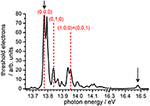Figure 1. TPEPICO spectrum of CO2 recorded using the CERISES set-up at the DESIRS beamline of the SOLEIL synchrotron. Black solid arrows indicate the photon energies (Ephot) at which reactivity studies in the DC mode have been performed, while red dashed lines indicate the energies chosen for experiments in the coincidence mode, i.e., with selective internal excitation of the ${\text{CO}}_{2}^{+}$ cation (see section Results and Discussion).

Following the assignment of Liu et al. (2000a), the photoelectron band at Ephot = 13.78 eV corresponds to the transition ${\text{CO}}_{2}^{+}$(0,0,0) ${\text{X}}^{2}{\Pi }_{\text{g},3/2}$ ← CO2(0,0,0) ${\text{X}}^{1}{\Sigma }_{\text{g}}^{+}$, hence producing the ${\text{CO}}_{2}^{+}$ cation in its electronic ground state with no vibrational excitation, hereafter indicated as (0,0,0). The band at Ephot = 13.84 eV corresponds to one of the four vibronic components of the transition ${\text{CO}}_{2}^{+}$(0,1,0) ${\text{X}}^{2}{\Pi }_{\text{g}}$ ← CO2(0,0,0) X1$\sum _{\text{g}}^{+}$, more precisely either the ${{}^{2}\Delta }_{5/2}$ or 2Σ+ component that we cannot distinguish at our limited resolution (see Liu et al., 2000a paper for the attribution of the four components), thus generating the ${\text{CO}}_{2}^{+}$ cation in its electronic ground state with one quantum of vibrational excitation in the bending ν2 mode, hereafter indicated as (0,1,0). The band at Ephot = 13.95 eV corresponds to the overlap of two transitions, namely ${\text{CO}}_{2}^{+}$(1,0,0) X ${{}^{2}\prod }_{\text{g},1/2}$ ← CO2(0,0,0) X ${{}^{1}\Sigma }_{\text{g}}^{+}$ and ${\text{CO}}_{2}^{+}$(0,0,1) X ${{}^{2}\prod }_{\text{g},3/2}$ ← CO2(0,0,0) ${\text{X}}^{1}{\Sigma }_{\text{g}}^{+}$, and therefore leads to ${\text{CO}}_{2}^{+}$ in the X state with one quantum of vibrational excitation in either the symmetric (ν1) or the antisymmetric (ν3) stretching vibration, hereafter indicated as (1,0,0) + (0,0,1).

A sufficient number of ion counts on the threshold electron signal have been observed to perform reactivity experiments with the state-selected cations at the three photon energies (red lines in Figure 1) corresponding to the vibronic bands giving ${\text{CO}}_{2}^{+}$ with either no or low vibrational excitation. The intensity of other vibronic bands corresponding to higher internal excitation of ${\text{CO}}_{2}^{+}$ was not sufficient to study reactivity in coincidence. For this reason, we decided to perform some measurements not in the coincidence mode, i.e., without pure state-selection but with parent ions in a distribution of excitation that can vary with the photon energy. Two photon-energies were chosen: at Ephot = 13.48 eV, corresponding to the ${\text{CO}}_{2}^{+}$(0,0,0) ${\text{X}}^{2}{\Pi }_{\text{g},3/2}$ ← CO2(0,0,0) ${\text{X}}^{1}{\Sigma }_{\text{g}}^{+}$ transition, the ${\text{CO}}_{2}^{+}$ cation will be produced with no vibrational excitation; at Ephot = 16.48 eV, corresponding to a strong resonant autoionization transition via Rydberg states converging to the $\stackrel{~}{B}$ state of ${\text{CO}}_{2}^{+}$, the latter will be produced in the X electronic state but with a broad distribution of internal energies, hence in a mixture of low and high vibrational excitation (see Baer and Guyon, 1986 for details).

Deuterated methane (CD4) was used for the reactivity study, to avoid partial mass overlap between the strong parent ion peak at m/z 44 and the one due to the product of the H-atom transfer process at m/z 45. CD4 pressure in the scattering cell was kept in the range 1–2 × 10−4 mbar throughout the experiments. Some considerations on possible isotope effects arising when CD4 is replaced by CH4 are addressed in the Conclusions.

## Results and Discussion

### Results in the DC Mode

As already mentioned in the previous section, in the DC mode we have measured absolute values of the cross sections as a function of the collision energy ECM at two selected photon energies: Ephot = 13.78 eV (no vibrational excitation of the ${\text{CO}}_{2}^{+}$ cation) and Ephot = 16.48 eV (some excitation to high vibrational levels). The main reaction products observed are due to the CT and deuterium-atom-transfer channels (1) and (2), respectively:

Also, small amounts of ${\text{CD}}_{3}^{+}$, and minimal amounts of CD3${\text{CO}}_{2}^{+}$ are detected and attributed to the following channels (3), (4) and (5):

Using literature values (taken from NIST Chemistry Webbook1, with the exception of some products as specified in the following) for the standard enthalpies of formations (ΔfH°) of reagents and products we can assess that both channels (1) and (2) are exothermic, while channels (3) and (4) are endothermic. In particular, the CT channel (1) has a reaction enthalpy ΔrH° = −1.17 eV, while ΔrH° for (2) is equal to −1.26 eV, assuming that HOCO+ has the structure of the hydroxyformyl cation (Holmes et al., 2006). The production of ${\text{CD}}_{3}^{+}$ can derive either from D transfer process (3) or from dissociative CT (4). In the former case, the DOCO radical might be formed in association with the methyl cation, and the overall process is calculated to be endothermic by about 0.56 eV, using the heat of formation of HOCO as reported in Francisco et al. (2010). In the latter case, the methyl cation derives from dissociation of the CT product and the process is endothermic by about 0.61 eV.

The CD3${\text{CO}}_{2}^{+}$ formation can be due to D loss from the ion-molecule adduct ${\text{CO}}_{2}^{+}$-CD4 (reaction 5). We can attempt to estimate the reaction enthalpy of (5) assuming that CD3${\text{CO}}_{2}^{+}$ has the structure of the methoxycarbonyl cation [an average value for its heat of formation ΔfH°(CH3OCO+) is 5.57±0.19 eV, as reported in Holmes et al., 2006] to get ΔrH° = −1.10 eV. We note in passing that the adduct ${\text{CO}}_{2}^{+}$-CD4 was not observed, as expected, as the CD4 pressure in the scattering cell used throughout the experiments (about 2 × 10−4 mbar) was too low to allow for secondary collisions for its stabilization.

Cross sections for products ${\text{CD}}_{4}^{+}$, DOCO+ and ${\text{CD}}_{3}^{+}$ as well as ${\text{CD}}_{5}^{+}$ (from secondary reactions of the primary ${\text{CD}}_{4}^{+}$ and DOCO+ products) measured as a function of the collision energy when the reagent ${\text{CO}}_{2}^{+}$ ion is in its ground vibrational state (i.e., at Ephot = 13.78 eV) are reported in Figure 2, while results for Ephot = 16.48 eV are shown in Figure 3. The cross section for ${\text{CD}}_{5}^{+}$ was measured to correct the absolute value of the cross section for reactions (1) and (2) due to product ion losses via the highly efficient secondary reactions operative at the deuterated methane pressures used:

with k = 1.1 × 10−9 cm3·molecule−1·sec−1 for reaction (6) (Anicich, 2003) and k = 7.2 × 10−10 cm3·molecule−1·sec−1 for reaction (7) (Anicich, 2003). The measured ${\text{CD}}_{5}^{+}$ yields have been redistributed among the ${\text{CD}}_{4}^{+}$ and DOCO+ products on the basis of the ${\text{CD}}_{4}^{+}$/DOCO+ yield ratio (that changes with collision energy from ~2 at low energies up to ~7 at high energies) and of the different rate coefficients for reactions (6) and (7). In Figures 2, 3 the uncorrected and corrected cross sections for ${\text{CD}}_{4}^{+}$ are labeled as “${\text{CD}}_{4}^{+}$” and “${\text{CD}}_{4}^{+}$ corr” respectively, and the same notation is used for DOCO+.

FIGURE 2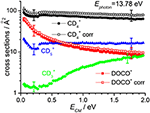Figure 2. Reactive cross sections for products ${\text{CD}}_{4}^{+}$ (uncorrected, black circles), DOCO+ (uncorrected, red squares), ${\text{CD}}_{3}^{+}$ (green diamonds), and ${\text{CD}}_{5}^{+}$ (blue triangles) measured as a function of the collision energy (ECM) in the DC mode at a photon energy Ephot = 13.78 eV. The open black circles and open red squares are the ${\text{CD}}_{4}^{+}$ and DOCO+ cross sections corrected to include the contribution of secondary reactions leading to ${\text{CD}}_{5}^{+}$. The dotted line represents the ${\text{CD}}_{4}^{+}$ cross sections corrected for the instrumental effect due to decreased collection efficiency at low ECM (see text for details). Error bars on all the data are about 30%: for the sake of clarity only two error bars are reported, at arbitrarily chosen low and high collision energy values.

FIGURE 3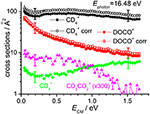Figure 3. Reactive cross sections for products ${\text{CD}}_{4}^{+}$ (uncorrected, black circles), DOCO+ (uncorrected, red squares), ${\text{CD}}_{3}^{+}$ (green diamonds), and CD3${\text{CO}}_{2}^{+}$ (magenta triangles) measured as a function of the collision energy (ECM) in the DC mode at a photon energy Ephot = 16.48 eV. The open black circles and open red squares are the ${\text{CD}}_{4}^{+}$ and DOCO+ cross sections corrected to include the contribution of secondary reactions leading to ${\text{CD}}_{5}^{+}$ (data not shown). The dotted line represents the ${\text{CD}}_{4}^{+}$ cross sections corrected for the instrumental effect due to decreased collection efficiency at low ECM (see text for details). Error bars on all the data are about 30%: for the sake of clarity only two error bars are reported, at arbitrarily chosen low and high collision energy values.

The sudden increase in the cross section shown for ${\text{CD}}_{4}^{+}$ and ${\text{CD}}_{5}^{+}$ products at ECM ~ 0.3 eV (clearly visible in the data of Figure 2, but also present in the data of Figure 3) is an instrumental effect due to a decrease in the collection efficiency for “slow” products. In particular, when product ions are produced at very low velocities in the lab frame, they have a chance to move backwards in the 1st octopole (O1) in the opposite direction from the parent ions, and they then face the last electrode before this octopole, L3, which is set to a potential of −0.4 V. As these ions are produced in O1, their fate depends on their initial kinetic energy and the relative values of the O1 and L3 potentials. At high collision energies, the mean potential of O1 is very low (negative values) and all product ions going back in O1 are reflected on L3 and later detected. At low collision energies, the O1 potential can be higher than that of L3, and backward product ions can be lost, accounting for the step in the product yield observed below collision energies of ~0.3 eV. To correct for such effect, we have rescaled the data measured at ECM ≤ 0.3 eV by a fixed multiplication factor, chosen equal to 1.12 to match the data measured at ECM < 0.3 eV with those measured at higher collision energy. Implicit in this way of rescaling data is the assumption that the number of product ions lost at low collision energy is independent on the collision energy. The corrected data (reported only for ${\text{CD}}_{4}^{+}$ cross sections corrected for the presence of secondary reactions leading to ${\text{CD}}_{5}^{+}$) are shown as dashed lines in Figures 2, 3.

According to the above-mentioned thermochemistry, the formation of ${\text{CD}}_{3}^{+}$ via either (3) or (4) is endothermic and the cross-section for its formation, when ${\text{CO}}_{2}^{+}$ is generated with no vibrational excitation (see green diamonds in Figure 2), shows the expected threshold behavior, with an appearance energy compatible with the endothermicity. Above threshold, the cross section increases accordingly with collision energy. The small amount of signal observed below the threshold is an artifact due to the tail of the very intense mass peak at 20 m/z (${\text{CD}}_{4}^{+}$). Data measured at Ephot = 16.48 eV show non-negligible cross sections even at low ECM, thus indicating that vibrational excitation of the ${\text{CO}}_{2}^{+}$ cation can promote the endothermic channel.

At Ephot = 13.78 eV and at the CD4 pressure used, the yield of CD3${\text{CO}}_{2}^{+}$ was below the detection limit, while at Ephot = 16.48 eV it was possible to measure a cross section for this very minor channel (data for CD3${\text{CO}}_{2}^{+}$ in Figure 3 are multiplied by 300 to be able to show them in the same scale of the other products).

In Table 2 results at the two photon energies are summarized by reporting the branching ratios (BRs) for the observed product channels and the energy-dependent rate constants. BR for the i-th channel have been calculated from the absolute cross sections according to the expression:

$BR(i)=σi∑σi$

The energy-dependent total rate constants ktot(Eave) have been estimated using the expression ktot(Eave) = 〈V〉 · σtot, were σtot is the total reaction cross section (i.e., ∑σi), as measured in this work (see data in Figures 3, 4) and < v> is the average relative velocity that can be estimated from the collision energy ECM (see Ervin and Armentrout, 1985 and Nicolas et al., 2002 for a more detailed treatment). While the total rate constants do not change (within the error bars) when increasing the amount of internal excitation of the ${\text{CO}}_{2}^{+}$ cation (compare the values at different photon energies but same Eave), a slight change in the BRs is observed when increasing the collision energy, which favors the production of ${\text{CD}}_{4}^{+}$ (and ${\text{CD}}_{3}^{+}$) over that of DOCO+.

TABLE 2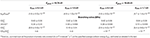Table 2. Energy-dependent rate constants and branching ratios (BRs) for the title reaction measured in the DC mode at two different values of photon energies (Ephot) and average collision energies (Eave).

FIGURE 4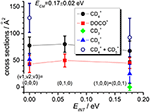Figure 4. Reactive cross sections for products ${\text{CD}}_{4}^{+}$ (uncorrected, black circles), DOCO+ (red squares), ${\text{CD}}_{3}^{+}$ (green diamonds), and ${\text{CD}}_{5}^{+}$ (blue triangles) measured as a function of the vibrational excitation of the ${\text{CO}}_{2}^{+}$ reagent (EINT) at a fixed collision energy ECM = 0.17 ± 0.02 eV. The open circles are the ${\text{CD}}_{4}^{+}$ cross sections corrected for the contribution of secondary reactions leading to ${\text{CD}}_{5}^{+}$. Lines connecting points are only a guide for the eyes.

Interestingly, our results for the branching ratios (see Table 2) show reaction (1) to be the dominant channel at all the explored collision energies, in net disagreement with some of the existing values for the branching ratios (see Table 1). As already mentioned, in fact, in the literature there is a spread of the branching ratios for reaction (1) and (2) ranging from 1:0 (Durup-Ferguson et al., 1983) to 0.6:0.4 (Smith et al., 1978), to 0.5:0.5 (Copp et al., 1982), to 0.28:0.72 (Tsuji et al., 1994) and 0.25:0.75 (Huntress et al., 1980), and finally to 0:1 (Harrison and Myher, 1967; Rakshit and Warneck, 1980). We provide here some explanations for the differences observed between our experiment and previous ones:

1. In addition to working with CD4, in our experiment we perform a mass selection of the parent ion before reaction. In this way, we eliminate the contribution due to the 13${\text{CO}}_{2}^{+}$ parent ion that appears at the same m/z as the HOCO+ product (from residual not fully deuterated methane) and represents ~1% of the parent ion intensity. Additionally, our experimental procedure consists in measuring both parent and product ion yields first with the target gas in the collision cell, secondly with the target gas in the chamber. In this way, we correct for any contribution of “impurities” coming from the source at the same mass as the product (namely 13${\text{CO}}_{2}^{+}$/HOCO+). In one of the earlier papers (Tsuji et al., 1994), the experiment is performed without parent ion mass selection, and no correction for 13${\text{CO}}_{2}^{+}$ is mentioned. Hence the claimed HOCO+ branching ratio (0.72) is most likely overestimated. In Durup-Ferguson et al. (1983), the parent ion mass selection is performed, although no indication is given about the mass resolution. In Rakshit and Warneck (1980), a mixing of ${\text{CO}}_{2}^{+}$, CO2${\text{CO}}_{2}^{+}$ and H2O+ parent ions are used. The values from Huntress et al. (1980) are given without any experimental details, for which a reference is given to an earlier paper (Huntress, 1977) where the ICR set-up is described. However, the earlier paper does not contain data for the title reaction, and it is impossible to infer whether and how the 13${\text{CO}}_{2}^{+}$ contribution was taken into account.

2. In our experiment, we keep the target gas pressure as low as possible to limit the number of secondary reactions. Some of the earlier works (Harrison and Myher, 1967; Kasper and Franklin, 1972; Tsuji et al., 1994) have a pressure in the reaction cell higher than ours by a factor 30-50, with about the same cell length. Such differences may lead to underestimating the BR for ${\text{CH}}_{4}^{+}$ if secondary reactions are not adequately accounted for (as done in our study).

3. In our experiment, we use a pure target gas, while some of the earlier works perform mass spectrometry studies in mixtures of gases. If some CO2 is present in the region where ${\text{CH}}_{4}^{+}$ products are generated by the ${\text{CO}}_{2}^{+}$ + CH4 reaction, the ${\text{CH}}_{4}^{+}$ will be easily consumed by the efficient reaction ${\text{CH}}_{4}^{+}$ + CO2 → HOCO+ + CH3 (k = 1.2 × 10−9 cm3·molecule−1·s−1) that will produce HOCO+, leading to a negative bias in the ${\text{CH}}_{4}^{+}$/HOCO+ ratio. This is an issue in Rakshit and Warneck (1980) as highlighted by Copp et al. (1982), where CO2 is present in the reaction cell, as well as in Ryan and Harland (1974), where mixing of CH4 and CO2 occurs in the reaction region, in Tsuji et al. (1994), Harrison and Myher (1967) and Kasper and Franklin (1972).

4. In our experiments we mass select ionic products. Hence we can directly give BRs among different channels. In some of the flow/drift tube experiments, the reaction rate constants are measured by observing the decline of the primary ion signal upon addition of the neutral gas. For instance, in Durup-Ferguson et al. (1983) no mention is made about the mass detection of products, as if the authors have assumed the exclusive formation of ${\text{CH}}_{4}^{+}$ via non-dissociative CT, not considering the possibility that HOCO+ might be produced.

### Results in the TPEPICO Mode

In the TPEPICO mode, we recorded cross sections for the reaction of ${\text{CO}}_{2}^{+}$ ions in the (0,0,0) ground state and in two vibrationally excited states: (0,1,0) with one quantum of bending vibration and [(1,0,0) + (0,0,1)] corresponding to a combination of the symmetric and antisymmetric stretching vibration. Cross sections were measured for products ${\text{CD}}_{4}^{+}$, DOCO+ and ${\text{CD}}_{3}^{+}$ as well as ${\text{CD}}_{5}^{+}$ (from secondary reactions of the primary ${\text{CD}}_{4}^{+}$ products, see above). The ion yield for product CD3${\text{CO}}_{2}^{+}$ was too low to be detectable in coincidence. For most of the other products, reactive cross sections were measured at two different collision energies ECM = 0.17 ± 0.02 eV and 1.34 ± 0.01 eV and results are shown in Figures 4, 5. Despite the larger uncertainties (due to the low S/N ratio in the coincidence mode) cross section measurements are consistent with results obtained in the DC mode. In particular, when converting cross sections reported in Figure 4 for ${\text{CO}}_{2}^{+}$ at low collision energy and in the (0,0,0) ground state we obtain the following BRs: ${\text{CD}}_{4}^{+}$ (0.75 ± 0.25), DOCO+ (0.25 ± 0.11) and ${\text{CD}}_{3}^{+}$ (0.00 ± 0.03), entirely consistent, within the error bars, with the data obtained in the DC mode (see Table 2, second column from the left).

FIGURE 5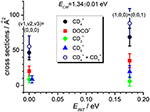Figure 5. Reactive cross sections for products ${\text{CD}}_{4}^{+}$ (uncorrected, black circles), DOCO+ (red squares), ${\text{CD}}_{3}^{+}$ (green diamonds), and ${\text{CD}}_{5}^{+}$ (blue triangles) measured as a function of the vibrational excitation of the ${\text{CO}}_{2}^{+}$ reagent (EINT) at a fixed collision energy ECM = 1.34 ± 0.01 eV. The open circles are the ${\text{CD}}_{4}^{+}$ cross sections corrected for the contribution of secondary reactions leading to ${\text{CD}}_{5}^{+}$.

In addition, the TPEPICO results confirm that cross sections for the exothermic CT (1) and deuterium-atom-transfer (2) channels change very little with increasing vibrational excitation, and the increase is more evident in data taken at high collision energy (Figure 5). Cross sections for the endothermic channel leading to ${\text{CD}}_{3}^{+}$ increase with collision energy but they do not seem to depend strongly on the internal excitation of the ionic parent, at least for the low internal excitations here explored (i.e., maximum one quantum of vibrational excitation).

The observed small dependence of reactions (1) and (2) from ${\text{CO}}_{2}^{+}$ vibrational excitation requires some consideration. First of all, we note that our result is in agreement with a previous study (Durup-Ferguson et al., 1983) in which a drift tube technique is used, and the dependence of the reaction rate constant on the internal energy of the ions is examined by varying the mass of the buffer gas. Despite the limitations of the technique compared to our truly state-selection method, in Durup-Ferguson et al. (1983) the non-dissociative CT giving ${\text{CH}}_{4}^{+}$ plus CO2 is found to occur at near the collision rate and to have little energy dependence and no measurable vibrational dependence.

We also note that, differently from the CH4 case, vibrational excitation of ${\text{CO}}_{2}^{+}$ ions was found to enhance the CT reaction probability with O2 (Durup-Ferguson et al., 1983; Ferguson et al., 1992; Viggiano and Morris, 1996; Nicolas et al., 2002). To rationalize such results, it should be considered that the two systems present several differences:

• CT channels have different exothermicities (−1.17 eV for CH4 and −1.71 for O2).

• The CT rate constant is substantially larger for CH4 (k = 0.3–1 × 10−9 cm3·molecule−1·s−1, see values in our Table 1) than for O2 (k ~ 5 × 10−11 cm3·molecule−1·s−1).

The inefficiency of the CT with O2 is attributed to the non-resonant character of the reaction, i.e., to the fact that Franck-Condon factors for O2 ionization at the ionization potential of CO2 (13.78 eV) are close to zero (see for instance Wacks, 1964). On the other hand, it is known that for CH4 the Franck-Condon factors are low at the ionization threshold and increase reaching a maximum in the region 13.5–14.5 eV (see for instance Stockbauer and Inghram, 1971). Thus, while in the CH4 case CT can occur efficiently at long range via the direct mechanism previously described, for O2 it should involve the formation of a collision complex. As tentatively explained and demonstrated in Ferguson et al. (1992), in the O2 case a small amount in the stretching excitation of ${\text{CO}}_{2}^{+}$ can lead to an increase in the CT probability. Hence, CH4 and O2 are quite different reaction systems, and in the latter case, the effect of ${\text{CO}}_{2}^{+}$ excitation could be more pronounced than for a reaction already at the Langevin limit (as in CH4).

More generally, the dynamics of CT processes is regulated by crossings among entrance and exit potential energy surfaces. When such crossings are occurring at distances for which the probability of electron transfer from one adiabatic PES to the other is unfavorable, the CT cross section will be very small even for exothermic processes (according to the Landau-Zener model for CT probability). The fact that increasing the vibrational excitation of the cation does not increase the CT cross section can be related to the fact that the crossing probability does not change much when increasing the vibrational excitation of ${\text{CO}}_{2}^{+}$, even though the exothermicity increases. Unfortunately, modeling the dynamics occurring on a ${\text{CO}}_{2}^{+}$- CH4 multidimensional potential energy surface which includes vibrational excitation of CH4 is not an easy task, and it is beyond the scope of our paper. We hope that our results will stimulate theoreticians and experts in ab-initio calculations to use this system as a test bench for theory.

## Conclusions

The reactivity of ${\text{CO}}_{2}^{+}$ with deuterated methane has been investigated experimentally by guided ion beam mass spectrometric techniques by changing either the kinetic energy of ${\text{CO}}_{2}^{+}$ or its vibrational excitation (using synchrotron radiation in the VUV energy range to produce vibrationally excited reagent ions). The main products are ${\text{CD}}_{4}^{+}$, DOCO+, and ${\text{CD}}_{3}^{+}$ and reactivity is found to depend on the reagent collision energy, but not so much on the vibrational excitation of ${\text{CO}}_{2}^{+}$.

An interesting issue is whether reaction rates and dynamics change or remain the same when CD4 is replaced by CH4. We do not expect charge transfer cross sections to be affected by a strong kinetic isotope effect (KIE). On the other hand, one can foresee a kinetic isotope effect in the H/D atom transfer channel leading to HOCO+/DOCO+. In particular, according to the semi-classical theory of primary KIE a normal effect (i.e., kH/kD > 1) is expected in the transfer of an H/D atom due to the vibrational zero-point energy differences for each of the vibrational modes of the reactants and transition state. Despite the limited mass resolution in our experimental set-up, we have managed to perform some tests using CH4 in the reaction cell and measuring BRs and cross sections at a fixed collision energy of 0.11 eV in the DC mode at a photon energy Ephot = 13.78 eV (i.e., same conditions of Figure 2). Products are observed at m/z values corresponding to ${\text{CH}}_{4}^{+}$, ${\text{CH}}_{5}^{+}$, and HOCO+. By correcting for secondary reactions (as detailed in the text) we obtain a BR of 0.74:0.26 = CH4+: HOCO+ and a total cross section of 199 (±30%) Å2, corresponding to an energy-dependent rate constant of (2.68 ± 0.8) × 10−9 cm3·molecule−1·s−1 to be compared with the CD4 value of (1.8 ± 0.5) × 10−9 at similar Eave (see our results in Table 2) and the BR of 0.63:0.36 = ${\text{CD}}_{4}^{+}$: DOCO+. This means a positive KIE kH/kD = 1.5(±0.6). On the other hand, our results show that when CD4 is replaced by the lighter isotopolog, the CT is more favored than the H atom transfer. This effect can be explained assuming that the different vibrational spacings in CD4/CH4 might change the Franck-Condon factors and the efficiencies of non-adiabatic transition probability among the entrance and exit potential energy surfaces. We note that in a similar reacting system CN+ plus CH4/CD4 a KIE in the total rate coefficient similar to the one observed in our case has been reported, namely kH/kD = 1.55 (±0.66) (Raksit et al., 1984).

To put our results into the context of plasma chemistry used for the conversion of CO2 in carbon-neutral fuels (Snoeckx et al., 2013; Snoeckx and Bogaerts, 2017), both the products (${\text{CH}}_{4}^{+}$ and HOCO+) of the reaction of ${\text{CO}}_{2}^{+}$ with CH4 eventually lead to the production of ${\text{CH}}_{5}^{+}$ and CH3, as shown in the following scheme:

Thus the energy initially used to ionize CO2 is transferred to CH4 to form ${\text{CH}}_{4}^{+}$, ${\text{CH}}_{5}^{+}$ and CH3. Only processes (2) and (9) lead to HOCO+ that, in addition to react with CH4, giving back CO2, can also recombine with electrons to yield CO plus OH.

## Data Availability

The datasets generated for this study are available on request to the corresponding author.

## Author Contributions

DA, CA, and PT contributed conception and design of the study. CA, CR, and JŽ planned and developed the experimental set-up. DA, CR, CA, AL, JŽ, MP, and CS contributed to data acquisition, data analysis, and interpretation of results. DA wrote the first draft of the manuscript. DA, PT, and CA wrote sections of the manuscript. All authors contributed to manuscript revision, read and approved the submitted version.

## Conflict of Interest Statement

The authors declare that the research was conducted in the absence of any commercial or financial relationships that could be construed as a potential conflict of interest.

## Acknowledgments

We thank the DESIRS beamline team, L. Nahon, G. Garcia, N. De Oliveira, and J.-F. Gil, for assistance during the synchrotron measurements and the technical staff of SOLEIL for running the facility under projects n° 20140033 and 20150468. CA and CR acknowledge the synchrotron SOLEIL for the support to the associated CERISES setup since 2008 and subsistence expenses during beamtime periods. DA acknowledges financial support from the EU-TNA program during beamtime n° 20140033. JŽ and MP acknowledge support from the Czech Science Foundation project No. 17-14200S. DA and PT thanks the Department of Physics of the University of Trento for support.

## Footnotes

1. ^NIST Chemistry WebBook, NIST Standard Reference Database Number 69 (2017), eds. P.J. Linstrom, and W.G. Mallard (Gaithersburg, MD: National Institute of Standards and Technology), 20899.

## References

Albritton, D. L. (1979). Energy dependences of ion-neutral reactions studied in drift tubes. In: Kinetics of Ion-Molecule Reactions, ed P. Ausloos (New York, NY: Plenum), 119.

Alcaraz, C., Nicolas, C., Thissen, R., Žabka, J., and Dutuit, O. (2004). 15N+ + CD4 and O+ + 13CO2 state-selected ion-molecule reactions relevant to the chemistry of planetary ionospheres. J. Phys. Chem. A 108, 9998–10009. doi: 10.1021/jp0477755

Alge, E., Villinger, H., and Lindinger, W. (1981). Drift tube investigations on the reactions of ${\text{O}}_{2}^{+}$ with CH4 and of ${\text{CO}}_{2}^{+}$ with NO in various buffer gases. Plasma Chem. Plasma Process 1, 65–71. doi: 10.1007/BF00566376

Anicich, V. G. (2003). An Index of the Literature for Bimolecular Gas Phase Cation-Molecule Reaction Kinetics, Report JPL-Publ-03–19.

Baer, M., and Guyon, P. M. (1986). Autoionization and isotope effect in the threshold photoelectron spectrum of 12CO2 and 13CO2. J. Chem. Phys. 85:4765. doi: 10.1063/1.451736

Bell, D. M., and Anderson, S. L. (2013a). Effects of collisional and vibrational velocity on proton and deuteron transfer in the reaction of HOD+ with CO. J. Phys. Chem. A 117, 1083–1093. doi: 10.1021/jp304208q

Bell, D. M., and Anderson, S. L. (2013b). Vibrationally enhanced charge transfer and mode/bond-specific H+ and D+ transfer in the reaction of HOD+ with N2O. J. Chem. Phys. 139:114305. doi: 10.1063/1.4820955

Bell, D. M., Howder, R. C., and Anderson, S. L. (2014). Effects of translational and vibrational excitation on the reaction of HOD+ with C2H2 and C2D2: mode- and bond-specific effects in exoergic proton transfer. J. Phys. Chem. A 118, 8360–8372. doi: 10.1021/jp501304v

Borodi, G., Luca, A., and Gerlich, D. (2009). Reactions of ${\text{CO}}_{2}^{+}$ with H, H2 and deuterated analogues. Int. J. Mass Spectr. 280, 218–225. doi: 10.1016/j.ijms.2008.09.004

Boyle, J. M., Bell, D. M., and Anderson, S. L. (2011). Reaction of C2${\text{H}}_{2}^{+}$ (n · ν2, m · ν5) with NO2: reaction on the singlet and triplet surfaces. J. Chem. Phys. 134:034313. doi: 10.1063/1.3517499

Candori, R., Pirani, F., Cappelletti, D., Tosi, P., and Bassi, D. (2003). State-to-state cross-sections for ${\text{N}}_{2}^{+}$(X,ν′ = 1,2) + Ar and Ar+(2Pj,mj) + N2(X,ν = 0) at low energies. Int. J. Mass Spectrom. 223–224, 499–506. doi: 10.1016/S1387-3806(02)00873-4

Chang, Y. C., Xu, Y., Lu, Z., Xu, H., and Ng, C. Y. (2012). Rovibrationally selected ion-molecule collision study using the molecular beam vacuum ultraviolet laser pulsed field ionization-photoion method: charge transfer reaction of ${\text{N}}_{2}^{+}$(X ${{}^{2}\Sigma }_{\text{g}}^{+}$; v+ = 0–2; N+ = 0–9) + Ar. J. Chem. Phys. 137:104202. doi: 10.1063/1.4750248

Chong, S. L., and Franklin, J. L. (1971). High-pressure ion–molecule reactions in carbon monoxide and carbon monoxide–methane mixtures. J. Chem. Phys. 54:1487. doi: 10.1063/1.1675043

Copp, N. W., Hamdan, M., Jones, J. D. C., Birkinshaw, K., and Twiddy, N. D. (1982). A selected ion flow tube study of the reactions of the gaseous ion ${\text{CO}}_{2}^{+}$ at 298 K. Chem. Phys. Lett. 88, 508–511. doi: 10.1016/0009-2614(82)83164-3

Cunha de Miranda, B., Romanzin, C., Chefdeville, S., Vuitton, V., Žabka, J., Polášek, M., et al. (2015). Reactions of state-selected atomic oxygen ions O+(4S, 2D, 2P) with methane. J. Phys. Chem. A 119, 6082–6098. doi: 10.1021/jp512846v

Derai, R., Kemper, P. R., and Bowers, M. T. (1985). Effect of reactant ion internal and translational energy on the rate constants of the charge exchange reactions: ${\text{CO}}_{2}^{+}+$O2${\text{O}}_{2}^{+}$ +CO2 and ${\text{O}}_{2}^{+}+$O2 → O2+${\text{O}}_{2}^{+}$. J. Chem. Phys. 82:4517. doi: 10.1063/1.448706

Durup-Ferguson, M., Böhringer, H., Fahey, D. W., and Ferguson, E. E. (1983). Enhancement of charge-transfer reaction rate constants by vibrational excitation at kinetic energies below 1 eV. J. Chem. Phys. 79:265. doi: 10.1063/1.445566

Ervin, K. M., and Armentrout, P. B. (1985). Translational energy dependence of Ar++XY → ArX+ + Y (XY = H2, D2, HD) from thermal to 30 eV CM. J. Chem. Phys. 83, 166–189. doi: 10.1063/1.449799

Ferguson, E. E., Van Dorenai, J. M., Viggiano, A. A., Morris, R. A., Paulson, J. F., Stewart, J. D., et al. (1992). Internal and translational energy effects on the charge- transfer reaction of ${\text{CO}}_{2}^{+}$ with O2. Int. J. Mass Spectr. Ion Proc. 117, 261–282. doi: 10.1016/0168-1176(92)80098-L

Francisco, J. S., Muckerman, J. T., and Yu, H. G. (2010). HOCO radical chemistry. Acc. Chem. Res. 43, 1519–1526. doi: 10.1021/ar100087v

Harrison, A. G., and Myher, J. J. (1967). Ion-molecule reactions in mixtures with D2 or CD4. J. Chem. Phys. 46:3276. doi: 10.1063/1.1841209

Holmes, J., Aubry, C., and Mayer, P. (2006). Assigning Structures to Ions in Mass Spectrometry. Boca Raton, FL: CRC Press; Taylor & Francis Group.

Huntress, W. T. Jr. (1977). Laboratory studies of bimolecular reactions of positive ions in interstellar clouds, in comets, and in planetary atmospheres of reducing composition. Astrophys. J.Suppl. Ser. 33, 495–514. doi: 10.1086/190439

Huntress, W. T. Jr., McEwan, M. J., Karpas, Z., and Anicich, V. (1980). Laboratory studies of some of the major ion-molecule reactions occurring on cometary comae. Astrophys. J.Suppl. Ser. 44, 481–488. doi: 10.1086/190701

Kasper, S. F., and Franklin, J. L. (1972). Ion-molecule reactions in the system CO2-CH4. J. Chem. Phys. 56:1156. doi: 10.1063/1.1677338

Liu, J., Chen, W., Hsu, C.-W., Hochlaf, M., Evans, M., Stimson, S., et al. (2000a). High resolution pulsed field ionization–photoelectron study of ${\text{CO}}_{2}^{+}$(${\text{X}}^{2}{\Pi }_{\text{g}}$) in the energy range of 13.6–14.7 eV. J. Chem. Phys. 112, 10767–10777. doi: 10.1063/1.481721

Liu, J., Hochlaf, M., and Ng, C. Y. (2000b). Pulsed field ionization–photoelectron bands for (A 2Πu and B 2${\Sigma }_{\text{u}}^{+}$) in the energy range of 17.2–19.0 eV: an experimental and theoretical study. J. Chem. Phys. 113:7988. doi: 10.1063/1.1314354

Martini, L. M., Lovascio, S., Dilecce, G., and Tosi, P. (2018). Time-resolved CO2 dissociation in a nanosecond pulsed discharge. Plasma Chem. Plasma Proc. 38, 707–718. doi: 10.1007/s11090-018-9893-3

Matta, M., Withers, P., and Mendillo, M. (2013). The composition of Mars' topside ionosphere: effects of hydrogen. J. Geophys. Res. Space Phys. 118, 2681–2693. doi: 10.1002/jgra.50104

Merkt, F., Mackenzie, S. R., Rednall, R. J., and Softley, T. P. (1993). Zero kinetic energy photoelectron spectrum of carbon dioxide. J. Chem. Phys. 99:8430. doi: 10.1063/1.466212

Nahon, L., de Oliveira, N., Garcia, G. A., Gil, J.-F., Pilette, B., Marcouillé, O, Lagarde, B., and Polack, F. (2012). DESIRS: a state-of-the-art VUV beamline featuring high resolution and variable polarization for spectroscopy and dichroism at SOLEIL. J. Synchrotron Rad. 19, 508–520. doi: 10.1107/S0909049512010588

Nicolas, C., Alcaraz, C., Thissen, R., Žabka, J., and Dutuit, O. (2002). Effects of ion excitation on ion-molecule reactions of the Mars, Venus, and Earth ionospheres. Plan. Space Sci. 50, 877–887. doi: 10.1016/S0032-0633(02)00063-6

Rakshit, A. B., and Warneck, P. (1980). Reactions of ${\text{CO}}_{2}^{+}$, CO2${\text{CO}}_{2}^{+}$ and H2O+ ions with various neutral molecules. J. Chem. Soc. Faraday Trans. 2 76, 1084–1092. doi: 10.1039/F29807601084

Raksit, A. B., Schiff, H. I., and Bohme, D. K. (1984). A selected ion flow tube study of the kinetics of CN+ reactions at 296 ± 2 K. Int. J. Mass Spectrom. and Ion Proc. 56, 321–335. doi: 10.1016/0168-1176(84)85058-2

Ryan, K. R., and Harland, P. W. (1974). Ionic collision process in mixtures of methane with carbon dioxide and nitrous oxide. Int. J. Mass Spectrom. Ion Proc. 15:197.

Scapinello, M., Martini, L. M., Dilecce, G., and Tosi, P. (2016). Conversion of CH4 /CO2 by a nanosecond repetitively pulsed discharge. J. Phys. D: Appl. Phys. 49:075602. doi: 10.1088/0022-3727/49/7/075602

Smith, D., Adams, N. G., and Miller, T. M. (1978). A laboratory study of the reactions of N+, ${\text{N}}_{2}^{+}$, ${\text{N}}_{3}^{+}$, ${\text{N}}_{4}^{+}$, O+, ${\text{O}}_{2}^{+}$, and NO+ ions with several molecules at 300 K. J. Chem. Phys. 69, 308–318. doi: 10.1063/1.436354

Snoeckx, R., Aerts, R., Tu, X., and Bogaerts, A. (2013). Plasma-based dry reforming: a computational study ranging from the nanoseconds to seconds time scale. J. Phys. Chem. C 117, 4957–4970. doi: 10.1021/jp311912b

Snoeckx, R., and Bogaerts, A. (2017). Plasma technology – a novel solution for CO2 conversion? Chem. Soc. Rev. 46, 5805–5863. doi: 10.1039/C6CS00066E

Stockbauer, R., and Inghram, M. G. (1971). Experimental relative franck-condon factors for the ionization of methane, ethane, and propane. J. Chem. Phys. 54, 2242–2246. doi: 10.1063/1.1675158

Tenewitz, J. E., Lê, T., Martinez, O. Jr., Ard, S. G., Shuman, N. S., Sanchez, J. C., et al. (2018). Kinetics of CO+ and ${\text{CO}}_{2}^{+}$ with N and O atoms. J. Chem. Phys. 148:084305. doi: 10.1063/1.5011195

Tsuji, M., Matsumura, K., Funatsu, T., Nishimura, Y., and Obase, H. (1994). Ion/molecule reactions of ${\text{CO}}_{2}^{+}$ with simple aliphatic hydrocarbons at thermal energy. Int. J Mass Spectr. Ion Proc. 135, 165–180. doi: 10.1016/0168-1176(94)03996-8

Viggiano, A. A., and Morris, R. A. (1996). Rotational and vibrational energy effects on ion–molecule reactivity as studied by the VT-SIFDT technique. J. Phys. Chem. 100, 19227–19240. doi: 10.1021/jp962084x

Wacks, M. E. (1964). Franck-condon factors for the ionization of CO, NO, and O2. J. Chem. Phys. 41, 930. doi: 10.1063/1.1726035

Wang, L., Yi, Y., Wu, C., Guo, H., and Tu, X. (2017). One-step reforming of CO2 and CH4 into high-value liquid chemicals and fuels at room temperature by plasma-driven catalysis. Angew. Chem. Int. Ed. 56, 13679–13683. doi: 10.1002/anie.201707131

Keywords: vibrational excitation, plasma, astrochemistry, Mars atmosphere, synchrotron radiation, ion-molecule reaction, CO2 dissociation

Citation: Ascenzi D, Romanzin C, Lopes A, Tosi P, Žabka J, Polášek M, Shaffer CJ and Alcaraz C (2019) State-Selected Reactivity of Carbon Dioxide Cations (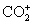) With Methane. Front. Chem. 7:537. doi: 10.3389/fchem.2019.00537

Received: 25 February 2019; Accepted: 15 July 2019;
Published: 02 August 2019.

Edited by:

Ramesh L. Gardas, Indian Institute of Technology Madras, India

Reviewed by:

Aparna Shastri, Bhabha Atomic Research Centre (BARC), India
Naved I. Malek, Sardar Vallabhbhai National Institute of Technology Surat, India

Copyright © 2019 Ascenzi, Romanzin, Lopes, Tosi, Žabka, Polášek, Shaffer and Alcaraz. This is an open-access article distributed under the terms of the Creative Commons Attribution License (CC BY). The use, distribution or reproduction in other forums is permitted, provided the original author(s) and the copyright owner(s) are credited and that the original publication in this journal is cited, in accordance with accepted academic practice. No use, distribution or reproduction is permitted which does not comply with these terms.

*Correspondence: Daniela Ascenzi, daniela.ascenzi@unitn.it

Present address: Christopher J. Shaffer, Sherwin Williams, VAST R&D, Minneapolis, MN, United States